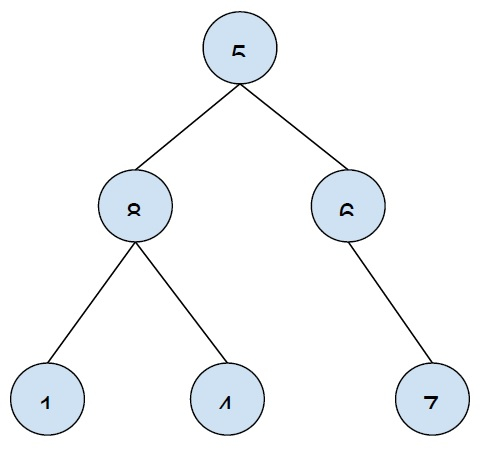# Print all leaf nodes of an n-ary tree using DFS in C++

In this problem, we are given a 2-D array containing the edge of an n-ary where edge defines the edge of the n-ary tree. We have to print all the leaf nodes of the created a-ary tree.

The n-ary tree is a tree with has maximum n children i.e. a node can have 1, 2, ...n child nodes.

Let’s see an example to understand the problem −

Input: edge[][] = {{5,8}, {5,6}, {8,1}, {8,4}, {6,7}}
Output: 1 4 7

Explanation − let's create a tree using the edge array −The leaf nodes of this tree are 1, 4, 7.

To solve this problem, we will traverse the tree using DFS (it will find the leaf node of every subtree). Also, visited nodes of the array are marked. If the node has a child (if not leaf node), we will flag the value and print nodes without a child node.

## Example

This program shows the implementation of our solution −

Live Demo

#include <bits/stdc++.h>
using namespace std;
void DFS(list<int> t[], int node, int parent) {
int flag = 0;
for (auto ir : t[node]) {
if (ir != parent) {
flag = 1;
DFS(t, ir, node);
}
}
if (flag == 0)
cout<<node<<"\t";
}
int main() {
list<int> t;
pair<int, int> edges[] = {
{ 1, 2 },
{ 1, 3 },
{ 2, 4 },
{ 3, 5 },
{ 3, 6 },
{ 3, 7 },
{ 6, 8 }
};
int cnt = sizeof(edges) / sizeof(edges);
int node = cnt + 1;
for (int i = 0; i < cnt; i++) {
t[edges[i].first].push_back(edges[i].second);
t[edges[i].second].push_back(edges[i].first);
}
cout<<"Leaf nodes of the tree are:\n";
DFS(t, 1, 0);
return 0;
}

## Output

Leaf nodes of the tree are −
4 5 8 7